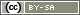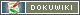This translation is older than the original page and might be outdated. See what has changed.

# Use Selected Items in Another Question

Occasionally, a question should not show all items, but only those previously selected in one of the other questions. Plus, as may be the case, the number of items should be restricted as well.

This chapter explains how to use items in another question. Usually, 2-3 lines of PHP code are enough to do this. If you have not used PHP code yet, please read Introduction to PHP Code before proceeding.

The following question (TF04) is used as an example for this chapter: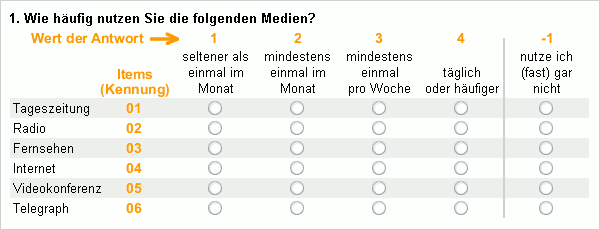In the following question (TF05), only media used at least once a month by the respondent should be available for selection.

Tip: It is important that both questions contain the same items with the same IDs. The simplest way to achieve this is by copying the question (Duplicate Question function in the question above) and then changing the Question Type in the copy and the text where applicable.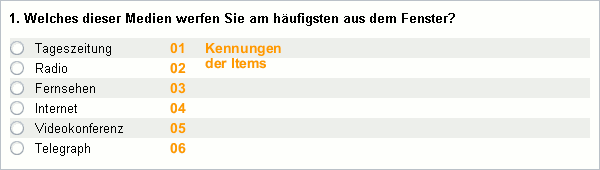The objective now is that the second question only shows the media (items) for selection that were given at least a score of 2 in the first question.

### Solution in a Nutshell

The function `getItems()` provides you with a list of items used at least (min) once a month (value of 2). You can transfer this list with the function question() – but of course only if it contains at least one element.

In order for the PHP code to work, the question TF04 must be asked on a previous page in the questionnaire.

```\$media = getItems('TF04', 'min', 2);
if (count(\$media) > 0) {
question('TF05', \$media);
}```

The rest of the manual describes how you work with arrays in detail. This is particularly interesting if `getItems()` does not help.

### The Long Way

The function `getItems()` creates an array – this can be done the long way. An array just has to be created that has all the item IDs which should appear in the second question. It works like this:

```\$itemlist = array(); // create an empty list
\$number_items = 6; // check 6 items
\$question = 'TF04';

for (\$i=1; \$i<=\$number_items; \$i++) { // enumerate from 1 to 6
id(\$question.'_'., \$i); // gives e.g. TF_04_1TF04_01\$
// Does the item have a score of at least 2?
if (value(\$id) >= 2) {
\$itemlist[] = \$i;
}
}
// for information purposes only
html( '<p>'. count(\$itemlist).' relevant items: '. implode(', ', \$itemlist). '</p>' );
// proceed to the next page when none are used
if (count(\$itemlist) == 0) {
goToPage('next');
}
// ask second question with these items
question('TF05', \$itemlist);```

When programming these filters do not forget it can be possible that no items are selected at all. Therefore, in the above example, the participant is forwarded directly to the next page if he does not use a medium at least once a month.

The following information could be given for the example above: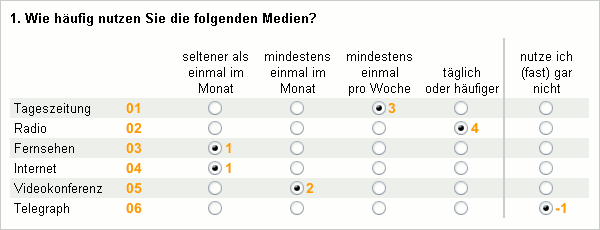Therefore, television, internet and telegraph do not meet the requirement of being used once a month. Page 2 would then look as follows: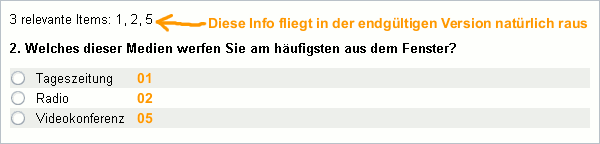Polarity profiles should now carried out for the individual types of media. However, only for media used at least once a month by the respondent. Furthermore, a maximum of 2 polarity profiles should be carried out. If the respondent uses more types of media then two should be selected at random.

6 similar questions (TF06 to TF11), in which only the question title varies, form the basis of this. “Daily newspaper” in the first question; “radio” in the next and so on. The question is created once, then copied five times and, finally, the name and question title is changed.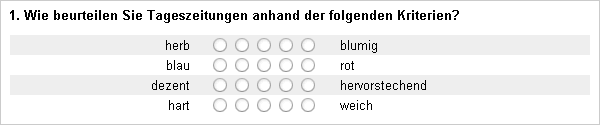How to create a list of media used is already described in detail above.

```// Option A: Conveniently
\$itemlist = getItems('TF04', 'min', 2); // list of relevant items```
```// Option B: The Long Way
\$itemlist = array(); // create an empty list
\$number_items = 6; // check 6 items
\$question = 'TF04';

for (\$i=1; \$i<=\$number_items; \$i++) {
\$id = \$question.'_'.\$i; // gives e.g. TF_04_1
if (value(\$id) >= 2) {
\$itemlist[] = \$i;
}
}```

If the list has more than two entries, then two entries have to be chosen at random. In order to achieves this, the list can be shuffled and then the first two entires copied into a new array:

```// ...
// continuation of option I
if (count(\$itemlist) > 2) {
shuffle(\$itemlist);
\$new_list = array();
\$new_list[] = \$itemlist; // copies element 0
\$new_list[] = \$itemlist; // copies element 1
} else {
\$new_list = \$itemlist; // otherwise use the list as always
}```

To save copying into a new list; simply shuffle the list and, afterwards, only use the first two entries.

```// ...
// continuation of option II
shuffle(\$itemlist);```

The relevant questions now have to be asked. The fastest way to do so is by also using an array. In an array, it is possible to specify which entry receives which index. An array is then created as follows: each index is stated, then an equals sign and a greater than symbol (`=>`), and only after then comes the actual entry. The result is then referred to as an associative array, as “normal” arrays are indexed.

```// ...
// continuation
\$question = array(
1 => 'TF06',
2 => 'TF07',
3 => 'TF08',
4 => 'TF09',
5 => 'TF10',
6 => 'TF11'
);```

In this array, the indices begin with 1, rather than 0. The array looks as follows:

 Index Value 1 2 3 4 5 6 TF_06 TF_07 TF_08 TF_09 TF_10 TF_11

The ID of the question belonging to item 2 (“radio”) is obtained using `\$question`, i.e. 'TF07'. Thus, questions regarding the type of media used can be asked very simply:

```// ...
// continuation
\$number = count(\$itemlist); // this many elements can be asked
if (\$number == 0) {
goToPage('next'); // None used? Then continue!
}
if (\$number > 2)  {
\$number = 2; // carry out a maximum of two polarity profiles
}
for (\$i=0; \$i<\$number; \$i++) {
\$item_ID = \$itemlist[\$i]; // one of the services used (1 to 6)
\$question_ID = \$questions[\$item_ID];
}```

The loop only asks a maximum of `\$number` questions. Previously, `\$number` was limited to always being a maximum of 2. At the same time, the number can never be greater than the number of media used (i.e. the length of the list).

The full code for this example is as follows:

```\$itemlist = getItems('TF04', 'min', 2);  // determine relevant items
shuffle(\$itemlist); // shuffle list
// define questions regarding items
\$questions = array(
1 => 'TF06',
2 => 'TF07',
3 => 'TF08',
4 => 'TF09',
5 => 'TF10',
6 => 'TF11'
);
\$number = count(\$itemlist); // this many elements can be asked
if (\$number == 0) {
goToPage('next'); // None used? Then continue!
}
if (\$number > 2) {
\$number = 2; // carry out a maximum of two polarity profiles
}
for (\$i=0; \$i<\$number; \$i++) {
\$item_ID = \$itemlist[\$i]; // one of the services used (1 to 6)
\$question_ID = \$questions[\$item_ID];  // the corresponding question
}```

This could look as follows in the questionnaire:Page 2 randomly shows the questions for newspaper, radio or video conference. Please note, that the order of the questions can be different to that of the items (above).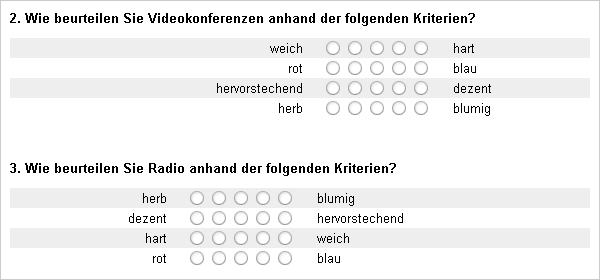Sometimes, the use is collected in different questions. For example, (selection) question NU01 asks about the use of web blogs, NU02 about the use of podcasts and NU03 for wikis.

In question TF13, items 1 to 3 should always be asked. Items 4, 5 and 6 should only be asked if the corresponding service is used. This certainly does not pose a problem after reading this manual:

```// First of all, the three questions are asked on page 1
question('NU01'); // Do you use web blogs? (1=no, 2=yes)
question('NU02'); // Do you use podcasts? (1=no, 2=yes)
question('NU03'); // Using wikis - item 2 is the use // Werte: 1=never 2=rarely 3=often```
```// An item list is created and queried on page 2
\$items = array(1,2,3); // items 1-3 are always asked
if (value('NU01') == 2) {
\$items[] = 4; // web blogs used => item 4
}
if (value('NU02') == 2) {
\$items[] = 5; // podcasts used => item 5
}
if (value('NU03_02') >= 2) {
\$items[] = 6; // wikis used at least rarely => item 6
}
question('TF13', \$items);```

The issue that selected items should be used from another question seems almost trivial – and in addition, another option “Other” should be shown.

To do so, another item “Other” is put in the second question (e.g. TF05) after the items from the filter question (TF04). The following example assumes that this item has the ID 13. The selected items are now determined and the number 13 is added to the list. Empty square brackets (`[]`) are used to do so - alternatively, the PHP command `array_push()` can be used here.

```\$selected = getItems('TF04'); // determine selected items
\$selected[] = 13; // add the number 13 to the list
question('AF05', \$selection); // show the follow-up question incl. item 13 ```

The ID of the additional item has to be entered in order for this filter to work. Certainly, identifying the items present in the second question, but not in the first, could be done first of all. The function `array_diff()` returns a list of these list elements and by using the function `array_merge()`, the lists can then be merged.

```// Determine additional items
\$items1 = getItems('TF04', 'all'); // list all items in question 1
\$items2 = getItems('TF05', 'all'); // list all items in question 2
\$added = array_diff(\$items2, \$items1); // items present in question 2 but not in question 1

\$auswahl = getItems('TF04'); // determine selected items